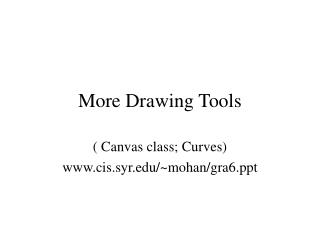# More Drawing Tools - PowerPoint PPT PresentationDownload PresentationMore Drawing Tools

More Drawing Tools
Download Presentation## More Drawing Tools

- - - - - - - - - - - - - - - - - - - - - - - - - - - E N D - - - - - - - - - - - - - - - - - - - - - - - - - - -
##### Presentation Transcript

1. More Drawing Tools ( Canvas class; Curves) www.cis.syr.edu/~mohan/gra6.ppt

2. Canvas class • Provides simple methods for creating screen window, establishing world window, mapping them, and simple Turtle graphics routines (moveto, lineto,…) • Supporting classes: • Point2: public Point2(), Point2(xx,yy), set(xx,yy), getX(), getY(), draw(); private x,y • IntRect, RealRect: publicInt/RealRect()/(ll,rr,bb,tt), set(ll,rr,bb,tt), draw(); private l,r,b,t

3. Canvas.h • public Canvas(w,h,title), setWindow(l,r,b,t), setViewport(l,r,b,t), getViewport(), getWindow(), getWindowAspectRatio(), clearScreen(), setBackgroundColor(r,g,b), setColor(r,g,b), lineTo(x,y)/(p), moveTo(x,y)/(p) • private CP, viewport, window

4. Using the Canvas class • Create a global object cvswhich initializes and opens the desired screen window. • Callback functions such as display access and invoke cvs.methods. • All initialization is done in the Canvas constructor • main() sets the drawing and background colors, registers the display() callback function, and enters the main event loop.

5. Implementing the Canvas class • Canvas constructor: invokes glutInit, glutInitDisplayMode, glutInitWindowSize, glutInitWindowPosition, glutCreateWindow, setWindow, setViewport, and CP.set, using default values for all parameters. • Other functions invoke appropriate gl... and glu… functions, e.g., Fig.3.28.

6. Other functions; turtle graphics • moveRel(dx, dy) • lineRel(dx, dy) • turnTo(angle) • turn(angle) //counterclockwise • forward(dist, isVisible)//invoking lineTo and moveTo, conditional on isVisible These functions make it easy to build complex drawings from simple ones.

7. Examples: using turtle graphics • Ex.3.5.2, hook motif, its repetitions varying starting point CP and initial direction CD • Ex.3.5.3, Polyspirals, iterating forward(len, 1); turn(angle); len += increment; • results shown in Fig.3.35 • Meander patterns • Drawing fractals, using F,L,R notation to abbreviate forward(d,1), turn(60), turn(-60)

8. Figures based on polygons • “Simple” polygon: only adjacent edges touch • “Regular” polygon: simple, equal sides, and adjacent sides meet at equal interior angles; implemented by iterating for i=0..n-1 to draw vertices at (R cos (2πi/n), R sin (2πi/n)), e.g., cvs.forward(L,1); cvs.turn(60) for a hexagon • “Stellation”: Connecting every other vertex of a regular polygon • “Rosette”: Interconnecting all vertices of an r.p.

9. Shapes with arcs • Teardrop patterns: lines have the same slope as the circular arc at the intersection point • Pie charts, e.g., with one slice “exploded”

10. Parametric Forms Graphics shapes can be described in many ways, each with its own [dis]advantages: • Implicit form: F(x,y)=0 • easy to test whether a point is on/inside/outside • Explicit form: y=g(x) or x=h(y), if single-valued • Parametric form, use a new “time” parameter in terms of which each coordinate is expressed: x(t)=f(t), y(t)=g(t)

11. Examples of parametric 2D curves • Line from (A.x,A.y) to (B.x,B.y): x(t)=A.x +(B.x-A.x)t, y(t)= A.y+(B.y-A.y)t • Ellipse of half-width W and half-height H: x(t)=W cos(t), y(t)= H sin (t) • Parabola: x(t)=at*t, y(t)=2at • Hyperbola: x(t)=a sec(t), y(t)=b tan(t) To extract implicit form, we would have to eliminate the parameter t from the equations.

12. Drawing curves using parametric reps. • Vary t from lower to upper extreme, take samples x(t), y(t) at small intervals of t • For better appearance, use more samples where curve changes sharply with t • For relatively smooth curves, generate points on the fly at uniform intervals of t, instead of first storing them.

13. Supercurves • Superellipses, supercircles: the bulge, i.e., the exponent 2 in the usual equations is allowed to vary; outward bulge>1, inward bulge<1, rectangle for bulge=1. Used in traffic circles and decorative patterns. • Superhyperbola, similarly defined

14. Polar Forms • Use angle θ instead of parameter t, e.g., x= f(θ)cos (θ), y= f(θ)sin (θ), • circle: f(θ )=K • cardioid: f(θ )=K(1+cos (θ)) • Archimedean spiral: f(θ )=K θ • conic sections: f(θ )=1/(1± e cos (θ)) • logarithmic spiral: f(θ )= K exp(a θ), cuts all radial lines at constant angle α, where cot(α)=a; has the same shape for any change of scale

15. 3D Curves Parametric forms specified using three functions x(t), y(t), z(t), e.g., • Helix: x(t)=W cos(t), y(t)=H sin(t), z(t)= bt • Conical Helix: W=H=t • Toroidal spiral: winding a string about a torus x(t)=(a sin(ct) +b) cos(t), y(t)=(a sin(ct) +b) sin(t), z(t)= a cos (ct)